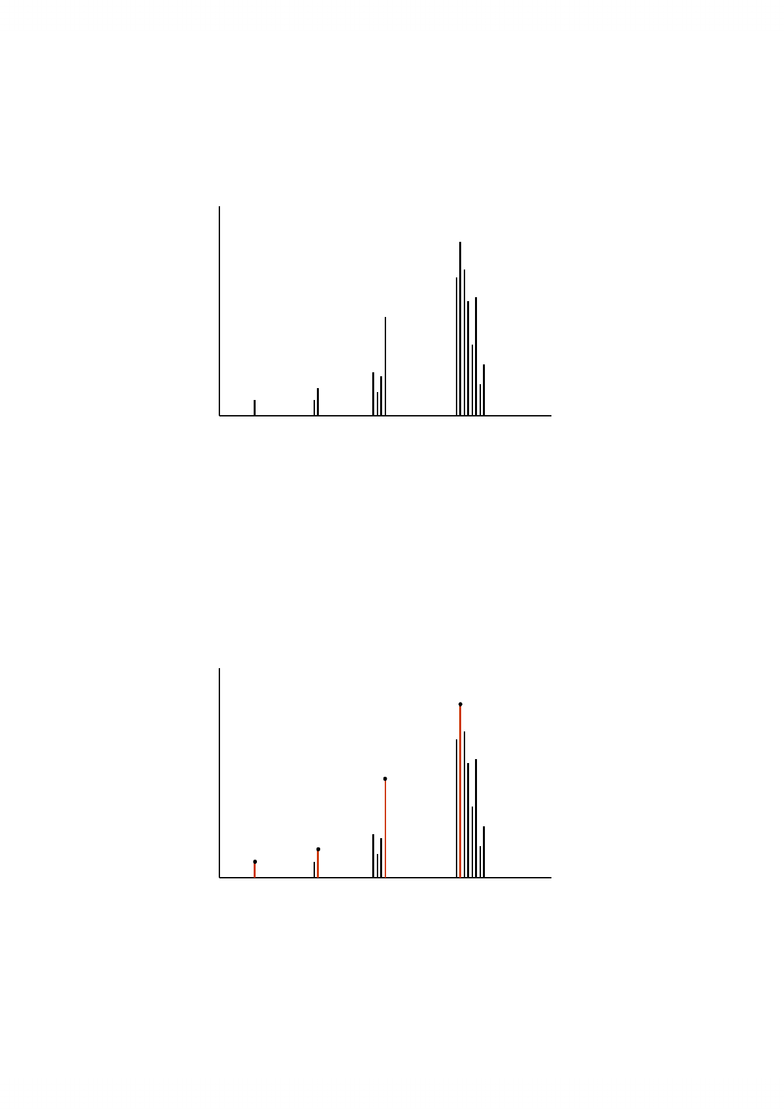Class Notes (1,200,000)
CA (650,000)
UW (20,000)
CS (1,000)
CS341 (20)
Lecture

# CS341 Lecture Notes - Intel 80386, Precautionary Statement, Quicksort

Department
Computer Science
Course Code
CS341
Professor
Forbes Burkowski

This preview shows pages 1-3. to view the full 20 pages of the document.1
Analysis of Algorithms
We design algorithms to solve problems.
What are valid inputs for the problem?
What output should we get for each input?
An algorithm is correct if for every input it finds
(computes) the correct output.
Running time.
The running time of algorithm Aon input xis the time
that algorithm Arequires to derive the correct output
given x.
We will denote this running time by RA(x).
We use order notation to describe running time.
Note: We did not mention running time of the program for A.
Running Time of an Instance
How to measure running time?
Wall-clock time is not a good measure, as it is
different from computer to computer, e.g. 80386
vs. Pentium III.
Hence we remove details of the computer (exact
speed of the processor, disk, memory, caching,
etc.).
We count the number of elementary operations
only.

Only pages 1-3 are available for preview. Some parts have been intentionally blurred.2
Running Time of an Instance (cont.)
An elementary operation is an operation whose time can
be bounded by a constant. It depends only on the
implementation of the operation (either in hardware or
software) and not on the inputs of the operation.
Examples:
simple arithmetic operations
simple comparisons
program flow control operations (if, goto)
Operations that are not elementary include:
maximum in an array of numbers
arithmetic operations or comparisons of multi-precision numbers
concatenation of two strings
• factorials
Beware: many programming languages (such as perl) offer
constructs that are not elementary operations
E.g. quicksort runs in O(n2)time
What it really means:
0
5
10
15
20
25
30
1 3 5 7 9 11 13 15
Order notation
running
time
n= size of input (in bits)

Only pages 1-3 are available for preview. Some parts have been intentionally blurred.3
Many inputs for a given size n
n
running time
inputs of
length one:
0, 1
inputs of
length two:
00, 01, 10, 11
inputs of length
three:
000, 001, …, 111
Worst case measure
n
running time
For each input size we select the worst case